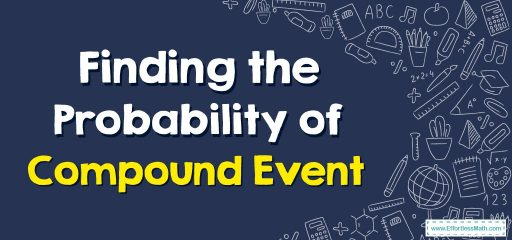# How to Find the Probability of Compound Event?

Compound probability is the probability of two or more independent events occurring together. In this article, you will learn how to find the probability of a compound event in a few simple steps.## A step-by-step guide tofinding the probability of a compound event

The compound probability of compound events (mutually inclusive or mutually exclusive) can be defined as the probability of two or more independent events occurring together. An independent event is an event whose outcome is not affected by the outcomes of other events.

A mutually inclusive event involves a situation where one event cannot occur with the other while an exclusive mutual event is when both events cannot occur at the same time. The compound probability will always lie between $$0$$ and $$1$$.

### Compound probability formulas

There are two formulas for calculating compound probability depending on the type of events that occur.In general, to find the compound probability, the probability of the first event is multiplied by the probability of the second event, and so on. The compound probability formulas are given below:

Mutually exclusive events compound the probability

$$\color{blue}{P(A\: or B) = P(A) + P(B)}$$

Using set theory, this formula is presented as follows:

$$\color{blue}{P\left(A\:∪\:B\right)\:=\:P\left(A\right)\:+\:P\left(B\right)}$$

Mutually inclusive events compound the probability

$$\color{blue}{P\left(A\:or\:B\right)\:=\:P\left(A\right)\:+\:P\left(B\right)\:-\:P\left(A\:and\:B\right)}$$

$$\color{blue}{P\left(A\:∪\:B\right)\:=\:P\left(A\right)\:+\:P\left(B\right)\:-\:P\left(A\:⋂\:B\right)}$$

where $$A$$ and $$B$$ are two independent events, and $$\color{blue}{P\left(A\:and\:B\right)\:=\:P\left(A\right)\times \:P\left(B\right)}$$

### Finding the Probability of Compound Event – Example 1:

If a dice is rolled, find the compound probability that either a $$2$$ or $$4$$ will be obtained.

Solution: $$P (2)=\frac {1}{6}$$, $$P(4)= \frac{1}{6}$$

Since this is an example of a mutually exclusive event, therefore, the compound probability formula is used: $$P\left(A\:or\:B\right)\:=\:P\left(A\right)\:+\:P\left(B\right)$$

$$P (2\: or\: 3)=\frac {1}{6}+\frac {1}{6}$$

$$=\frac {1}{3}$$

### What people say about "How to Find the Probability of Compound Event? - Effortless Math: We Help Students Learn to LOVE Mathematics"?

No one replied yet.

X
30% OFF

Limited time only!

Save Over 30%

SAVE $5 It was$16.99 now it is \$11.99